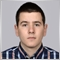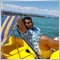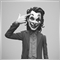5750

Hi guys, I have an EA that ive translated from mql4 , that doesnt trade. Ill send the code if anyone has ideas.

//+------------------------------------------------------------------+
//|                                                  Negative_EA.mq5 |
//|                        Copyright 2018, MetaQuotes Software Corp. |
//|                                             https://www.mql5.com |
//+------------------------------------------------------------------+
#property copyright "Copyright 2018, MetaQuotes Software Corp."
#property version   "1.00"

input double BarHeight1=1.0;
input double StopLoss1    = 150.0;
input double TakeProfit1  = 3.0;
input double BarHeight2=0;
input double StopLoss2    = 0;
input double TakeProfit2  = 0;
input double BarHeight3=0;
input double StopLoss3    = 0;
input double TakeProfit3  = 0;
input double BarHeight4=0;
input double StopLoss4    = 0;
input double TakeProfit4  = 0;
input double LotSize=0.1;

input int    MaxTrades  = 5;
input int    MagicNumber= 7827;

double TakeProfit,StopLoss;
datetime time1=0,time2=0;
int shift1,shift2;

double point=0;
int closetime,LotsDigit;
CSymbolInfo m_symbol;
datetime deal_time;
#define MA_MAGIC MagicNumber
//+------------------------------------------------------------------+
//| Expert initialization function                                   |
//+------------------------------------------------------------------+
int OnInit()
{
//---
//  if(MQLInfoInteger(MQL_TESTER) && IsDemoMQL4())

point=_Point;
// if(_Digits==3 || _Digits==5) point*=10;
if(SymbolInfoDouble(Symbol(),SYMBOL_VOLUME_MIN)==0.1) LotsDigit=1;
else if(SymbolInfoDouble(Symbol(),SYMBOL_VOLUME_MIN) == 0.01) LotsDigit = 2;
else if(SymbolInfoDouble(Symbol(),SYMBOL_VOLUME_MIN) == 1) LotsDigit=0;
minlot = NormalizeDouble(SymbolInfoDouble(Symbol(),SYMBOL_VOLUME_MIN),LotsDigit);
maxlot = NormalizeDouble(SymbolInfoDouble(Symbol(),SYMBOL_VOLUME_MAX),LotsDigit);
time1=TimeCurrent();

//-----
return INIT_SUCCEEDED;

//---
}
//+------------------------------------------------------------------+
//| Expert deinitialization function                                 |
//+------------------------------------------------------------------+
void OnDeinit(const int reason)
{
//---

}
//+------------------------------------------------------------------+
//| Expert tick function                                             |
//+------------------------------------------------------------------+
void OnTick()
{
MqlDateTime tm;
TimeToStruct(TimeCurrent(),tm);
//-----
MqlRates rt;
MqlTick last_tick;
SymbolInfoTick(_Symbol,last_tick);

Bid=last_tick.bid;
double Close[],Open[],High[],Low[];
ArraySetAsSeries(Close,true);
ArraySetAsSeries(Open,true);
CopyClose(_Symbol,_Period,0,3,Close);
CopyOpen(_Symbol,_Period,0,3,Open);
CopyHigh(_Symbol,_Period,0,3,High);
CopyLow(_Symbol,_Period,0,3,Low);

//--- go trading only for first ticks of new bar
if(CopyRates(_Symbol,_Period,0,2,rt)!=2)
{
Print("CopyRates of ",_Symbol," failed, no history");
return;
}
if(rt.tick_volume>1)
return;
//--- get current Moving Average
//--- check signals
ENUM_ORDER_TYPE signal=WRONG_VALUE;
time2=TimeCurrent();
shift1=iBarShift(Symbol(),0,time1);
shift2=iBarShift(Symbol(),0,time2);

//if(shift1!=shift2)
if(BarHeight1>0 && High-Low>=BarHeight1*_Point && Close>Open)Print("ok");OrderEntry(0,1);
if(BarHeight2>0 && High-Low>=BarHeight2*_Point && Close>Open)Print("ok");OrderEntry(0,2);
if(BarHeight3>0 && High-Low>=BarHeight3*_Point && Close>Open)Print("ok");OrderEntry(0,3);
if(BarHeight4>0 && High-Low>=BarHeight4*_Point && Close>Open)Print("ok");OrderEntry(0,4);

if(BarHeight1>0 && High-Low>=BarHeight1*_Point && Close<Open)Print("ok");OrderEntry(1,1);
if(BarHeight2>0 && High-Low>=BarHeight2*_Point && Close<Open)Print("ok");OrderEntry(1,2);
if(BarHeight3>0 && High-Low>=BarHeight3*_Point && Close<Open)Print("ok");OrderEntry(1,3);
if(BarHeight4>0 && High-Low>=BarHeight4*_Point && Close<Open)Print("ok");OrderEntry(1,4);

//---
}
//+------------------------------------------------------------------+

//+------------------------------------------------------------------+
//|                                                                  |
//+------------------------------------------------------------------+
bool IsDemoMQL4()
{
return(true);
else
return(false);
}
//+------------------------------------------------------------------+
//|                                                                  |
//+------------------------------------------------------------------+
int iBarShiftMQL4(string symbol,
int tf,
datetime time,
bool exact=false)
{
if(time<0) return(-1);
ENUM_TIMEFRAMES timeframe=TFMigrate(tf);
datetime Arr[],Time1;
CopyTime(symbol,timeframe,0,1,Arr);
Time1=Arr;
if(CopyTime(symbol,timeframe,time,Time1,Arr)>0)
{
if(ArraySize(Arr)>2) return(ArraySize(Arr)-1);
if(time<Time1) return(1);
else return(0);
}
else return(-1);
}
//+------------------------------------------------------------------+
double elots;
//+------------------------------------------------------------------+
//|                                                                  |
//+------------------------------------------------------------------+
void OrderEntry(int direction,int typei)
{

double tp=0,sl=0;
elots=LotSize;

if(typei==1)
{
TakeProfit=TakeProfit1;
StopLoss=StopLoss1;
}
if(typei==2)
{
TakeProfit=TakeProfit2;
StopLoss=StopLoss2;
}
if(typei==3)
{
TakeProfit=TakeProfit3;
StopLoss=StopLoss3;
}
if(typei==4)
{
TakeProfit=TakeProfit4;
StopLoss=StopLoss4;
}

{
if(direction==0)
{

sl,tp,"Louis EA");

}

if(direction==1)
{

if(StopLoss>0){sl=Bid+(StopLoss*_Point);}else{sl=0;}
if(TakeProfit>0){tp=Bid-(TakeProfit*_Point);}else{tp=0;}

sl,tp,"Louis EA");

}

}

}
//+------------------------------------------------------------------+
//|                                                                  |
//+------------------------------------------------------------------+
bool IsNewCandle()
{
static int BarsOnChart=0;
if(Bars(Symbol(),0)==BarsOnChart)
return (false);
BarsOnChart=Bars(Symbol(),0);
return(true);
}
//+------------------------------------------------------------------+
int OpenOrdersThisPair(string pair)
{
int total=0;
ulong ticket;
for(uint i=OrdersTotal()-1; i>=0;i--)
{
ticket=OrderGetTicket(i);
if(OrderSelect(ticket))
{
if(OrderGetString(ORDER_SYMBOL)==pair) total++;
}
}
return (total);
}
//+------------------------------------------------------------------+
//|                                                                  |
//+------------------------------------------------------------------+
ENUM_TIMEFRAMES TFMigrate(int tf)
{
switch(tf)
{
case 0: return(PERIOD_CURRENT);
case 1: return(PERIOD_M1);
case 5: return(PERIOD_M5);
case 15: return(PERIOD_M15);
case 30: return(PERIOD_M30);
case 60: return(PERIOD_H1);
case 240: return(PERIOD_H4);
case 1440: return(PERIOD_D1);
case 10080: return(PERIOD_W1);
case 43200: return(PERIOD_MN1);

case 2: return(PERIOD_M2);
case 3: return(PERIOD_M3);
case 4: return(PERIOD_M4);
case 6: return(PERIOD_M6);
case 10: return(PERIOD_M10);
case 12: return(PERIOD_M12);
case 16385: return(PERIOD_H1);
case 16386: return(PERIOD_H2);
case 16387: return(PERIOD_H3);
case 16388: return(PERIOD_H4);
case 16390: return(PERIOD_H6);
case 16392: return(PERIOD_H8);
case 16396: return(PERIOD_H12);
case 16408: return(PERIOD_D1);
case 32769: return(PERIOD_W1);
case 49153: return(PERIOD_MN1);
default: return(PERIOD_CURRENT);
}
}
//+------------------------------------------------------------------+18

we use an EA with MT4, or easier to use and easy to understand... really25095

Stanislav Ivanov:

Hi guys, I have an EA that ive translated from mql4 , that doesnt trade. Ill send the code if anyone has ideas.

you have 25 freelance job, but can't figure out why this code doesn't trade? hmm..5750